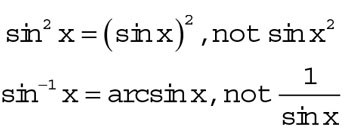##### Pre-Calculus Workbook For Dummies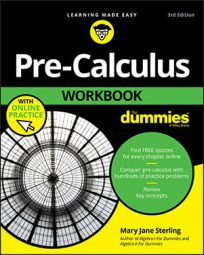Here are ten pitfalls that normally trip up the pre-calculus student. Make sure that you understand the order of operations, how to properly multiply binomials and monomials with exponents, how to break up and flip fractions, how not to combine terms, and how to keep from disregarding the negative sign.

## Going out of order (of operations)

Operations in an expression or an equation aren’t all meant to be done from left to right. For example, 3 – 7(x – 2) doesn’t equal –4(x – 2) or –4x + 8. You’re supposed to do multiplication first, which means distributing the –7 first 3 – 7(x – 2) = 3 – 7x + 14. And then you combine like terms to get –7x + 17.

Remember your order of operations (PEMDAS) all the time, every time:

Parentheses (and other grouping devices)

Exponents (and roots)

Multiplication and Division, from left to right in order as you find them

Addition and Subtraction, also from left to right

## Multiplying binomials incorrectly

When multiplying binomials, always remember to multiply them in the correct order. You remember FOIL — First, Outside, Inside, Last. This includes when squaring any binomial. The biggest mistake made in these situations is something like when squaring a binomial: (x – 4)2 and getting x2 + 16. That’s forgetting a whole lot of multiplying, and it’s not correct. It should look like this:

(x – 4)2 = x2 – 4x – 4x + 16 = x2 – 8x + 16.

You may use other orders, but it pays to be careful and consistent.

Don’t fall for a big trap and break a fraction up incorrectly.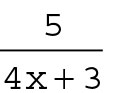doesn’t equal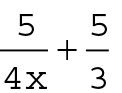.

If you’re skeptical, just pick a value for x and plug it into both expressions and see whether you get the same answer twice. You won’t, because it doesn’t work. On the other hand,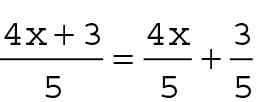.

See the difference?

When reducing fractions, each term in the fraction has to be divided. The division bar is a grouping symbol, and you have to simplify the numerator and denominator separately before doing the division. For example,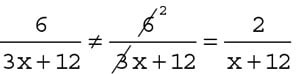.

Instead, you factor the denominator, first, and then reduce: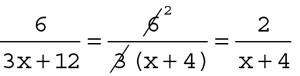## Combining terms that can’t be combined

Yet another frequent mistake occurs when terms that aren’t meant to be combined are combined. 4x – 1 suddenly becomes 3x, which it’s not. 4x – 1is simplified, meaning that it’s an expression that doesn’t contain any like terms. 3a4b5 + 2a5b4 is also simplified. (It can be factored, but it’s still simplified.) Those exponents are close, but close only counts in horseshoes and hand grenades. When counting in the real world, you can’t combine apples and bananas. Four apples plus three bananas is still four apples and three bananas. It’s the same in algebra: 4a + 3b is simplified.

## Forgetting to flip the fraction

When dealing with complex fractions, remember that you’re dealing with division of fractions.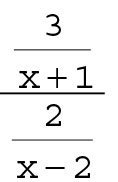doesn’t become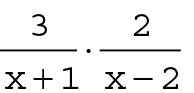.

Remember that a division bar is division. To divide a fraction, you must multiply by the reciprocal of the denominator, so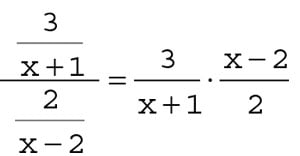.

## Losing the negative (sign)

It’s true that in life you’re not supposed to be negative, but in math, don’t disregard a negative sign — especially when subtracting polynomials or raising to powers.

(4x3 – 6x + 3) – (3x3 – 2x + 4) isn’t the same thing as 4x3 – 6x + 3 – 3x3 – 2x + 4. If you do it that way, you’re not subtracting the whole second polynomial, only its first term. The right way to do it is 4x3 – 6x + 3 – 3x3 – (–2x) – 4 = 4x3 – 6x + 3 – 3x3 + 2x – 4, which simplifies to x3 – 4x –1. The issue here is a special case of the failure to correctly apply the distributive law; it frequently occurs with other coefficients as well (not just –1). Failure to write the parentheses often directly contributes to these errors.

Similarly, when subtracting rational functions, take care of that negative sign.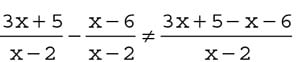What happened? Someone forgot to subtract the whole second polynomial on the top. Instead, this is the way to do it: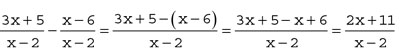And one other caution about negative signs is when raising negative expressions to powers. There's a difference between –26 and (–2)6. In the expression –26, the order of operations says to raise the 2 to the sixth power first, and then find the opposite: –26 = –64. The expression (–2)6 says to raise the number –2 to the sixth power: (–2)6 = 64.

## Oversimplifying roots

When it comes to roots, there are all kinds of errors that can occur. For instance,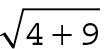suddenly becomes 5 when that’s not even close!

You know that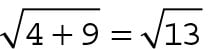.

Don’t add or subtract roots that aren’t like terms, either.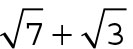isn’t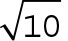, now or ever. They’re not like terms, so you can’t add them.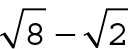?

You can rewrite it as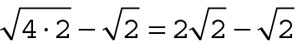and then subtract the like terms getting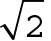.

## Executing exponent errors

When multiplying monomials, you don’t multiply the exponents.

x4 × x3 = x7, not x12. Don’t mistake the multiplication of exponential expressions with powers of exponential expressions.

Raising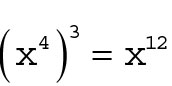.

Also, when there’s more than one term being raised to the power, you have to multiply the whole expression the number of times shown with the exponent. Raising (x2 – 2x +3)3 does not mean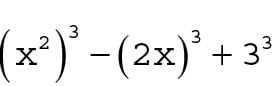.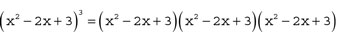.

## Ignoring extraneous

Sometimes, when solving radical or rational equations, you have to perform an operation (such as multiplying all the terms by a variable) which can introduce an extraneous or false answer.

For example, when told to solve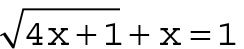you dutifully subtract x from each side and then square both sides of the equation: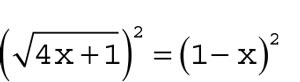or 4x + 1 = 1 – 2x + x2.

Simplifying, you get 0 = x2 – 6x = x(x – 6). This new equation has two solutions: x = 0 and x = 6. When substituting back into the original equation, the x = 0 works just fine: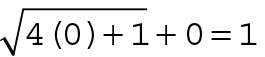becomes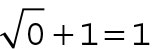.

But that’s not the case with x = 6: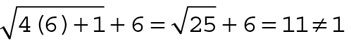The solution x = 6 is extraneous.

## Misinterpreting trig notation

The trig functions are great to work with, and they come with some specialty notation that makes writing about them quicker and easier. You just need to be careful and interpret the notation correctly.

The two cases in point have to do with squaring the function or finding the inverse of the function.

When you see sin2x, this means to find the sine and then square the result.